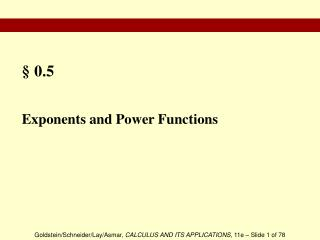DownloadDownload Presentation§ 0.5

# § 0.5

Télécharger la présentation## § 0.5

- - - - - - - - - - - - - - - - - - - - - - - - - - - E N D - - - - - - - - - - - - - - - - - - - - - - - - - - -
##### Presentation Transcript

1. §0.5 Exponents and Power Functions

2. Section Outline • Exponent Rules • Applications of Exponents • Compound Interest

3. Exponents

4. Exponents

5. Exponents

6. Exponents

7. Exponents

8. Applications of Exponents EXAMPLE Use the laws of exponents to simplify the algebraic expression. SOLUTION This is the given expression.

9. Applications of Exponents CONTINUED Subtract. Divide.

10. Compound Interest - Annual

11. Compound Interest - General

12. Compound Interest - General EXAMPLE (Quarterly Compound) Assume that a \$500 investment earns interest compounded quarterly. Express the value of the investment after one year as a polynomial in the annual rate of interest r. SOLUTION Since interest is not being compounded annually, we must use this formula. Replace P with 500, m with 4 (interest is compounded 4 times each year), and t with 1 (interest is being compounded for 1 year). Simplify.